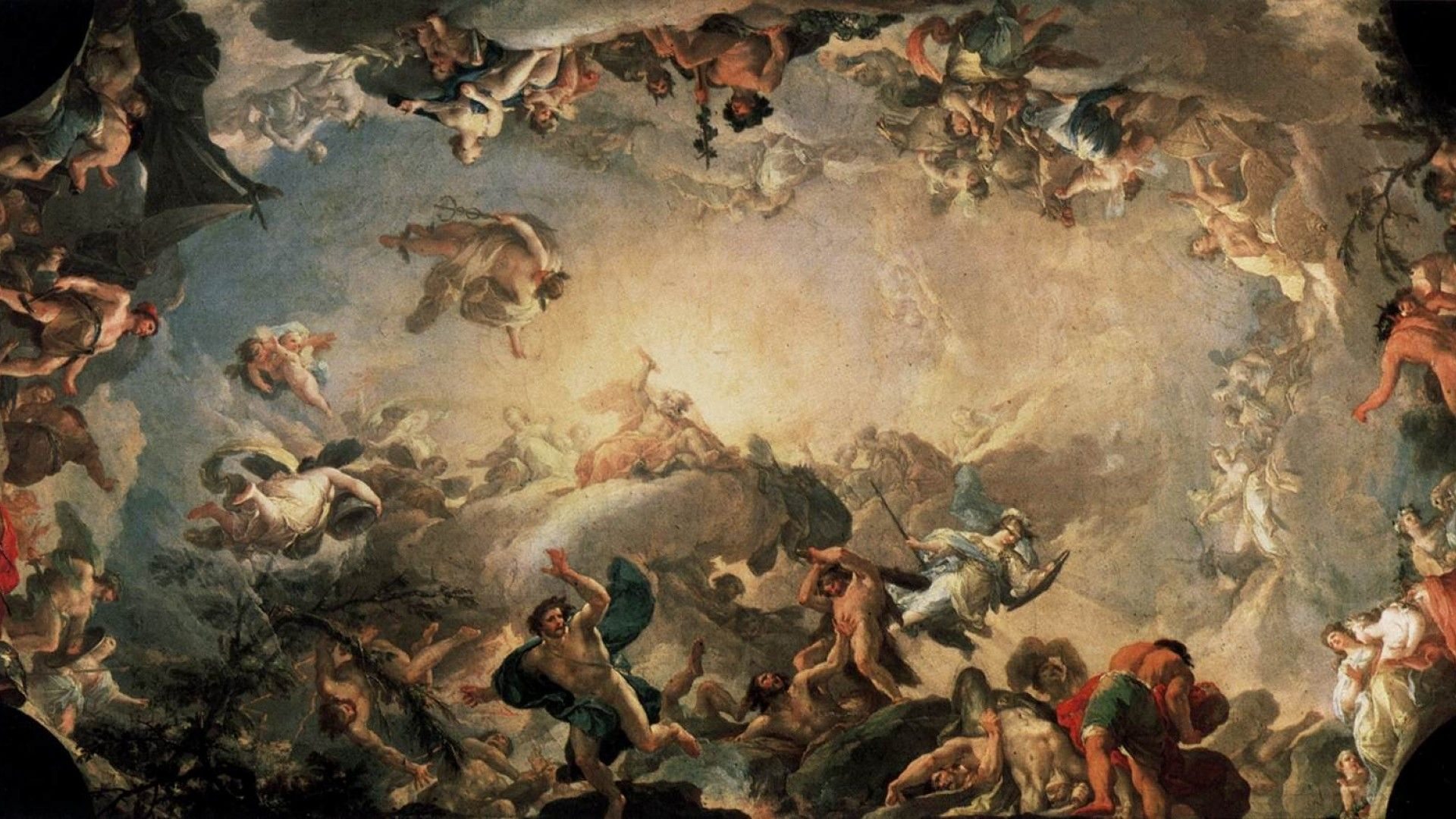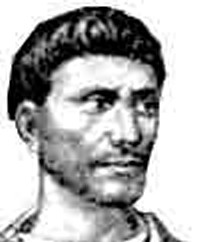TheDomeENTER THE WORLD OF MYTHS AND LEGENDS## Diophantus of AlexandriaDiophantus of Alexandria (Ancient Greek: Διόφαντος ὁ Ἀλεξανδρεύς; born probably sometime between AD 201 and 215; died aged 84, probably sometime between AD 285 and 299), sometimes called "the father of algebra", was an Alexandrian Greek mathematician and the author of a series of books called Arithmetica, many of which are now lost.

Diophantus of Alexandria, (flourished c. ad 250) Greek mathematician, famous for his work in algebra.

What little is known of Diophantus’s life is circumstantial. From the appellation “of Alexandria” it seems that he worked in the main scientific centre of the ancient Greek world; and because he is not mentioned before the 4th century, it seems likely that he flourished during the 3rd century. An arithmetic epigram from the Anthologia Graeca of late antiquity, purported to retrace some landmarks of his life (marriage at 33, birth of his son at 38, death of his son four years before his own at 84), may well be contrived. Two works have come down to us under his name, both incomplete. The first is a small fragment on polygonal numbers (a number is polygonal if that same number of dots can be arranged in the form of a regular polygon). The second, a large and extremely influential treatise upon which all the ancient and modern fame of Diophantus reposes, is his Arithmetica. Its historical importance is twofold: it is the first known work to employ algebra in a modern style, and it inspired the rebirth of number theory.

The Arithmetica begins with an introduction addressed to Dionysius—arguably St. Dionysius of Alexandria. After some generalities about numbers, Diophantus explains his symbolism—he uses symbols for the unknown (corresponding to our x) and its powers, positive or negative, as well as for some arithmetic operations—most of these symbols are clearly scribal abbreviations. This is the first and only occurrence of algebraic symbolism before the 15th century. After teaching multiplication of the powers of the unknown, Diophantus explains the multiplication of positive and negative terms and then how to reduce an equation to one with only positive terms (the standard form preferred in antiquity). With these preliminaries out of the way, Diophantus proceeds to the problems. Indeed, the Arithmetica is essentially a collection of problems with solutions, about 260 in the part still extant.

The introduction also states that the work is divided into 13 books. Six of these books were known in Europe in the late 15th century, transmitted in Greek by Byzantine scholars and numbered from I to VI; four other books were discovered in 1968 in a 9th-century Arabic translation by Qusṭā ibn Lūqā. However, the Arabic text lacks mathematical symbolism, and it appears to be based on a later Greek commentary—perhaps that of Hypatia (c. 370–415)—that diluted Diophantus’s exposition. We now know that the numbering of the Greek books must be modified: Arithmetica thus consists of Books I to III in Greek, Books IV to VII in Arabic, and, presumably, Books VIII to X in Greek (the former Greek Books IV to VI). Further renumbering is unlikely; it is fairly certain that the Byzantines only knew the six books they transmitted and the Arabs no more than Books I to VII in the commented version.

The problems of Book I are not characteristic, being mostly simple problems used to illustrate algebraic reckoning. The distinctive features of Diophantus’s problems appear in the later books: they are indeterminate (having more than one solution), are of the second degree or are reducible to the second degree (the highest power on variable terms is 2, i.e., x2), and end with the determination of a positive rational value for the unknown that will make a given algebraic expression a numerical square or sometimes a cube. (Throughout his book Diophantus uses “number” to refer to what are now called positive, rational numbers; thus, a square number is the square of some positive, rational number.) Books II and III also teach general methods. In three problems of Book II it is explained how to represent: (1) any given square number as a sum of the squares of two rational numbers; (2) any given non-square number, which is the sum of two known squares, as a sum of two other squares; and (3) any given rational number as the difference of two squares. While the first and third problems are stated generally, the assumed knowledge of one solution in the second problem suggests that not every rational number is the sum of two squares. Diophantus later gives the condition for an integer: the given number must not contain any prime factor of the form 4n + 3 raised to an odd power, where n is a non-negative integer. Such examples motivated the rebirth of number theory. Although Diophantus is typically satisfied to obtain one solution to a problem, he occasionally mentions in problems that an infinite number of solutions exists.

In Books IV to VII Diophantus extends basic methods such as those outlined above to problems of higher degrees that can be reduced to a binomial equation of the first- or second-degree. The prefaces to these books state that their purpose is to provide the reader with “experience and skill.” While this recent discovery does not increase knowledge of Diophantus’s mathematics, it does alter the appraisal of his pedagogical ability. Books VIII and IX (presumably Greek Books IV and V) solve more difficult problems, even if the basic methods remain the same. For instance, one problem involves decomposing a given integer into the sum of two squares that are arbitrarily close to one another. A similar problem involves decomposing a given integer into the sum of three squares; in it, Diophantus excludes the impossible case of integers of the form 8n + 7 (again, n is a non-negative integer). Book X (presumably Greek Book VI) deals with right-angled triangles with rational sides and subject to various further conditions.

The contents of the three missing books of the Arithmetica can be surmised from the introduction, where, after saying that the reduction of a problem should “if possible” conclude with a binomial equation, Diophantus adds that he will “later on” treat the case of a trinomial equation—a promise not fulfilled in the extant part.

Although he had limited algebraic tools at his disposal, Diophantus managed to solve a great variety of problems, and the Arithmetica inspired Arabic mathematicians such as al-Karajī (c. 980–1030) to apply his methods. The most famous extension of Diophantus’s work was by Pierre de Fermat (1601–65), the founder of modern number theory. In the margins of his copy of Arithmetica, Fermat wrote various remarks, proposing new solutions, corrections, and generalizations of Diophantus’s methods as well as some conjectures such as Fermat’s last theorem, which occupied mathematicians for generations to come. Indeterminate equations restricted to integral solutions have come to be known, though inappropriately, as Diophantine equations.



### Sources

 "Encyclopaedia Britannica" by Jacques Sesiano

### Our Mobile Application

Check out Our Mobile Application "Ancient Greece Reloaded"